# 双向 BFS 基本思路（含模板）以及两种「启发式搜索」算法## 题目描述

Tag : 「双向 BFS」、「启发式搜索」、「AStar 算法」、「IDAStar 算法」

输入：deadends = ["0201","0101","0102","1212","2002"], target = "0202"



输入: deadends = ["8888"], target = "0009"



输入: deadends = ["8887","8889","8878","8898","8788","8988","7888","9888"], target = "8888"



输入: deadends = ["0000"], target = "8888"



• 1 <= deadends.length <= 500
• target.length == 4

## 基本分析

「127. 单词接龙」定位困难，而本题定位中等。主要体现在数据范围上，思维难度上「127. 单词接龙」并不比本题难，大胆做。

「127. 单词接龙」原题链接在 这里，相关题解在 这里

## 双向 BFS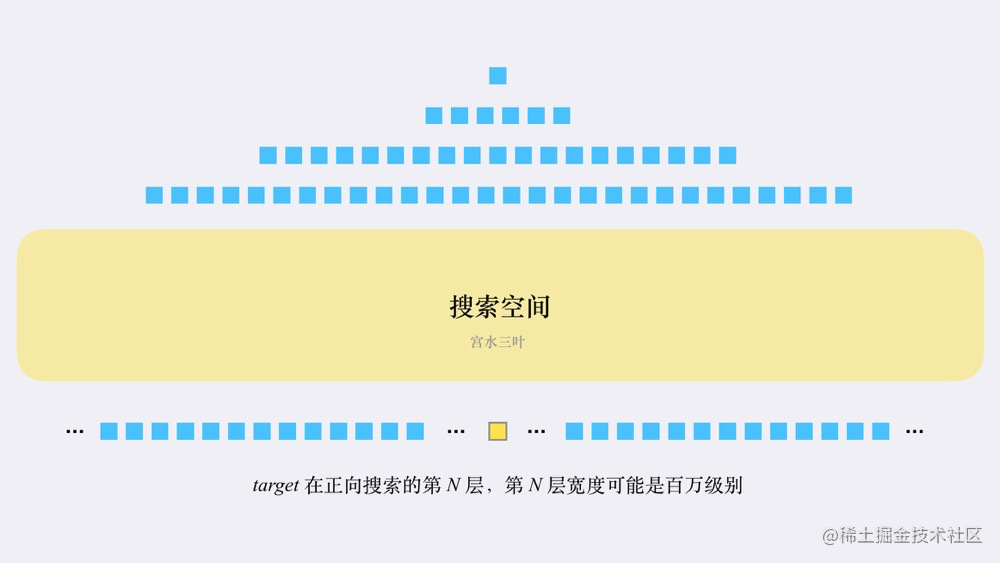「双向 BFS」 可以很好的解决这个问题：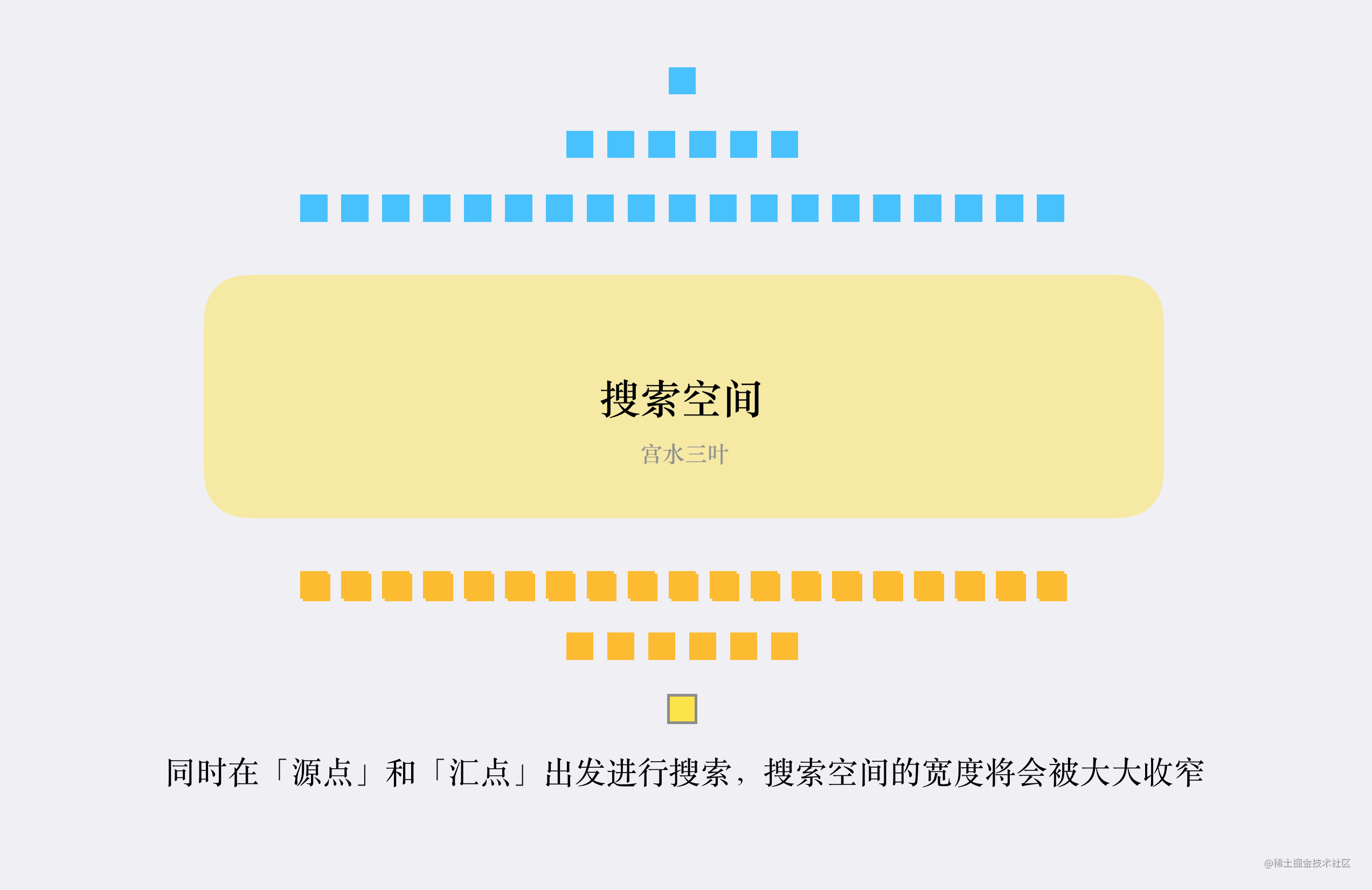「双向 BFS」的基本实现思路如下：

1. 创建「两个队列」分别用于两个方向的搜索；
2. 创建「两个哈希表」用于「解决相同节点重复搜索」和「记录转换次数」；
3. 为了尽可能让两个搜索方向“平均”，每次从队列中取值进行扩展时，先判断哪个队列容量较少；
4. 如果在搜索过程中「搜索到对方搜索过的节点」，说明找到了最短路径。

「双向 BFS」基本思路对应的伪代码大致如下：

d1、d2 为两个方向的队列
m1、m2 为两个方向的哈希表，记录每个节点距离起点的

// 只有两个队列都不空，才有必要继续往下搜索
// 如果其中一个队列空了，说明从某个方向搜到底都搜不到该方向的目标节点
while(!d1.isEmpty() && !d2.isEmpty()) {
if (d1.size() < d2.size()) {
update(d1, m1, m2);
} else {
update(d2, m2, m1);
}
}

// update 为从队列 d 中取出一个元素进行「一次完整扩展」的逻辑
void update(Deque d, Map cur, Map other) {}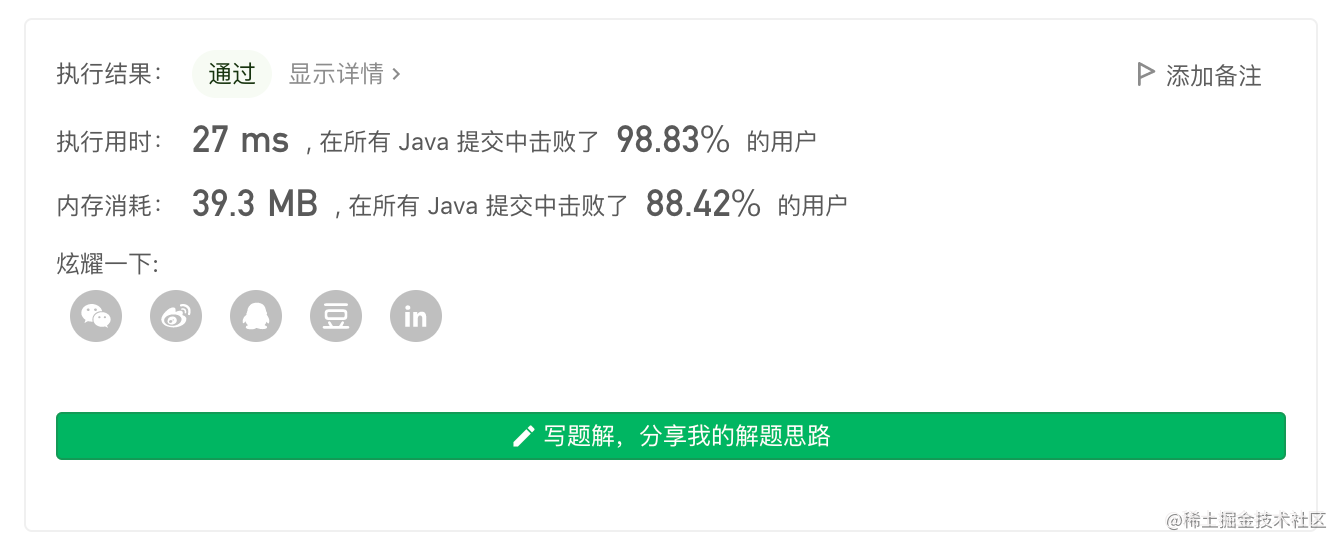class Solution {
String t, s;
Set<String> set = new HashSet<>();
public int openLock(String[] _ds, String _t) {
s = "0000";
t = _t;
if (s.equals(t)) return 0;
for (String d : _ds) set.add(d);
if (set.contains(s)) return -1;
int ans = bfs();
return ans;
}
int bfs() {
// d1 代表从起点 s 开始搜索（正向）
// d2 代表从结尾 t 开始搜索（反向）
Deque<String> d1 = new ArrayDeque<>(), d2 = new ArrayDeque<>();
/*
* m1 和 m2 分别记录两个方向出现的状态是经过多少次转换而来
* e.g.s
* m1 = {"1000":1} 代表 "1000" 由 s="0000" 替换 1 次字符而来
* m2 = {"9999":3} 代表 "9999" 由 t="9996" 替换 3 次字符而来
*/
Map<String, Integer> m1 = new HashMap<>(), m2 = new HashMap<>();
m1.put(s, 0);
m2.put(t, 0);

/*
* 只有两个队列都不空，才有必要继续往下搜索
* 如果其中一个队列空了，说明从某个方向搜到底都搜不到该方向的目标节点
* e.g.
* 例如，如果 d1 为空了，说明从 s 搜索到底都搜索不到 t，反向搜索也没必要进行了
*/
while (!d1.isEmpty() && !d2.isEmpty()) {
int t = -1;
if (d1.size() <= d2.size()) {
t = update(d1, m1, m2);
} else {
t = update(d2, m2, m1);
}
if (t != -1) return t;
}
return -1;
}
int update(Deque<String> deque, Map<String, Integer> cur, Map<String, Integer> other) {
String poll = deque.pollFirst();
char[] pcs = poll.toCharArray();
int step = cur.get(poll);
// 枚举替换哪个字符
for (int i = 0; i < 4; i++) {
// 能「正向转」也能「反向转」，这里直接枚举偏移量 [-1,1] 然后跳过 0
for (int j = -1; j <= 1; j++) {
if (j == 0) continue;

// 求得替换字符串 str
int origin = pcs[i] - '0';
int next = (origin + j) % 10;
if (next == -1) next = 9;

char[] clone = pcs.clone();
clone[i] = (char)(next + '0');
String str = String.valueOf(clone);

if (set.contains(str)) continue;
if (cur.containsKey(str)) continue;

// 如果在「另一方向」找到过，说明找到了最短路，否则加入队列
if (other.containsKey(str)) {
return step + 1 + other.get(str);
} else {
cur.put(str, step + 1);
}
}
}
return -1;
}
}


## AStar 算法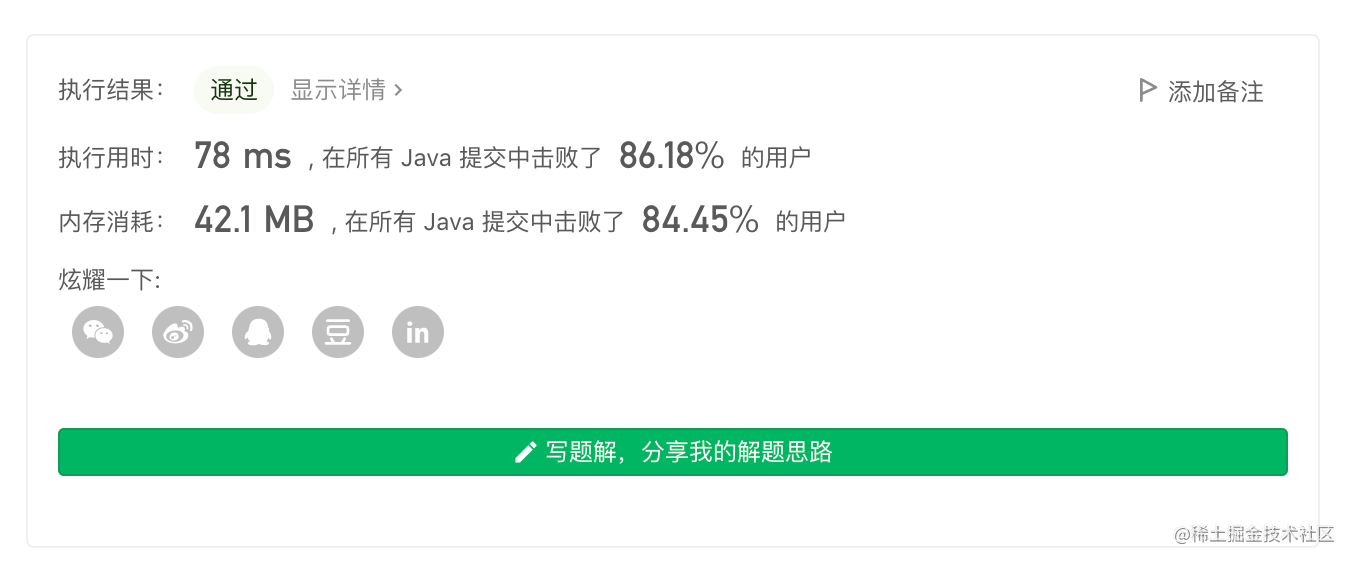class Solution {
class Node {
String str;
int val, step;
/**
*  str : 对应字符串
*  val : 估值（与目标字符串 target 的最小转换成本）
*  step: 对应字符串是经过多少步转换而来
*/
Node(String _str, int _val, int _step) {
str = _str;
val = _val;
step = _step;
}
}
int f(String str) {
int ans = 0;
for (int i = 0; i < 4; i++) {
int cur = str.charAt(i) - '0', target = t.charAt(i) - '0';
int a = Math.min(cur, target), b = Math.max(cur, target);
// 在「正向转」和「反向转」之间取 min
int min = Math.min(b - a, a + 10 - b);
ans += min;
}
return ans;
}
String s, t;
Set<String> set = new HashSet<>();
public int openLock(String[] ds, String _t) {
s = "0000";
t = _t;
if (s.equals(t)) return 0;
for (String d : ds) set.add(d);
if (set.contains(s)) return -1;

PriorityQueue<Node> q = new PriorityQueue<>((a,b)->a.val-b.val);
Map<String, Node> map = new HashMap<>();
Node root = new Node(s, f(s), 0);
map.put(s, root);
while (!q.isEmpty()) {
Node poll = q.poll();
char[] pcs = poll.str.toCharArray();
int step = poll.step;
if (poll.str.equals(t)) return step;
for (int i = 0; i < 4; i++) {
for (int j = -1; j <= 1; j++) {
if (j == 0) continue;
int cur = pcs[i] - '0';
int next = (cur + j) % 10;
if (next == -1) next = 9;

char[] clone = pcs.clone();
clone[i] = (char)(next + '0');
String str = String.valueOf(clone);

if (set.contains(str)) continue;
// 如果 str 还没搜索过，或者 str 的「最短距离」被更新，则入队
if (!map.containsKey(str) || map.get(str).step > step + 1) {
Node node = new Node(str, step + 1 + f(str), step + 1);
map.put(str, node);
}
}
}
}
return -1;
}
}


## IDA* 算法

• 仍然使用 f() 作为估值函数
• 利用旋转次数有限：总旋转次数不会超过某个阈值 $\max$
• 利用「迭代加深」的思路找到最短距离

PS. 上述的阈值分析是科学做法。对于本题可以利用数据弱，直接使用 $\max = 5 * 4$ 也可以通过，并且效果不错。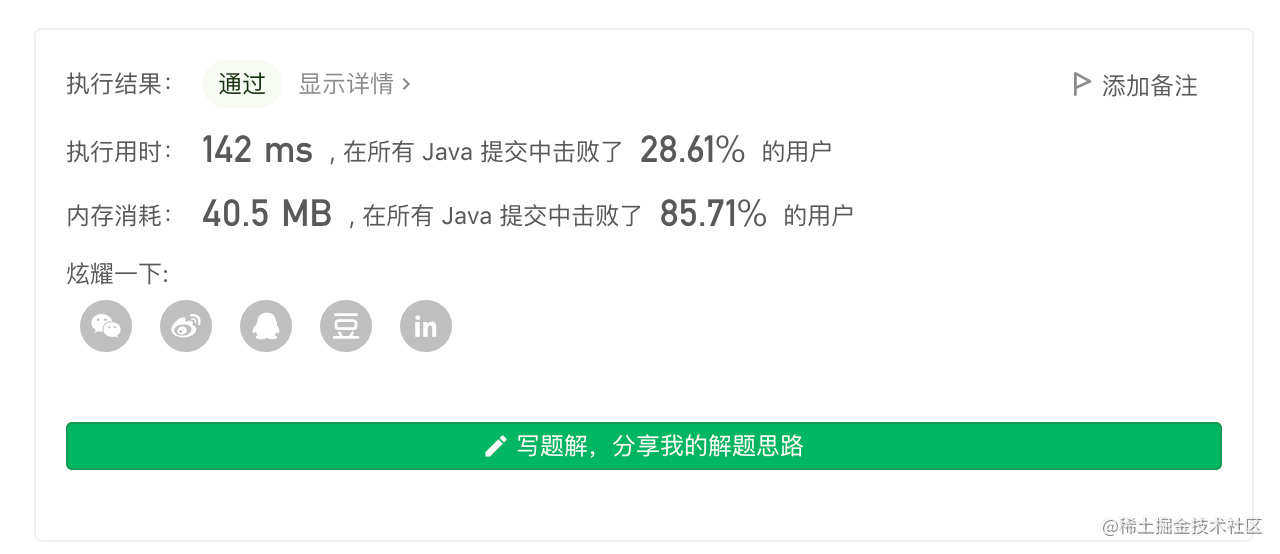class Solution {
String s, t;
String cur;
Set<String> set = new HashSet<>();
Map<String, Integer> map = new HashMap<>();
public int openLock(String[] ds, String _t) {
s = "0000";
t = _t;
if (s.equals(t)) return 0;
for (String d : ds) set.add(d);
if (set.contains(s)) return -1;

int depth = 0, max = getMax();
cur = s;
map.put(cur, 0);
while (depth <= max && !dfs(0, depth)) {
map.clear();
cur = s;
map.put(cur, 0);
depth++;
}
return depth > max ? -1 : depth;
}
int getMax() {
int ans = 0;
for (int i = 0; i < 4; i++) {
int origin = s.charAt(i) - '0', next = t.charAt(i) - '0';
int a = Math.min(origin, next), b = Math.max(origin, next);
int max = Math.max(b - a, a + 10 - b);
ans += max;
}
return ans;
}
int f() {
int ans = 0;
for (int i = 0; i < 4; i++) {
int origin = cur.charAt(i) - '0', next = t.charAt(i) - '0';
int a = Math.min(origin, next), b = Math.max(origin, next);
int min = Math.min(b - a, a + 10 - b);
ans += min;
}
return ans;
}
boolean dfs(int u, int max) {
if (u + f() > max) return false;
if (f() == 0) return true;
String backup = cur;
char[] cs = cur.toCharArray();
for (int i = 0; i < 4; i++) {
for (int j = -1; j <= 1; j++) {
if (j == 0) continue;
int origin = cs[i] - '0';
int next = (origin + j) % 10;
if (next == -1) next = 9;
char[] clone = cs.clone();
clone[i] = (char)(next + '0');
String str = String.valueOf(clone);
if (set.contains(str)) continue;
if (!map.containsKey(str) || map.get(str) > u + 1) {
cur = str;
map.put(str, u + 1);
if (dfs(u + 1, max)) return true;
cur = backup;
}
}
}
return false;
}
}